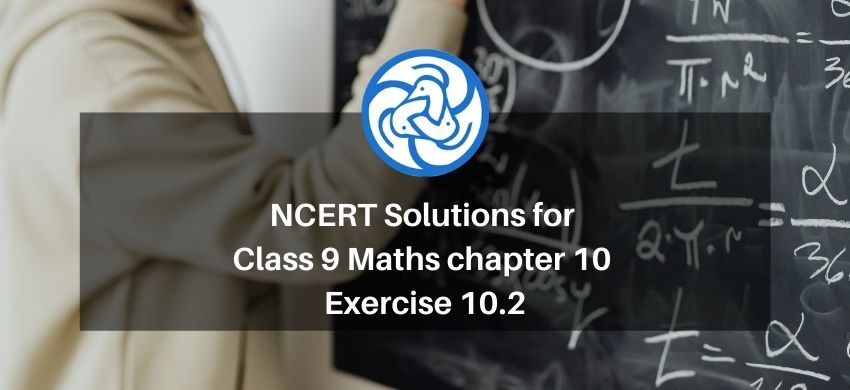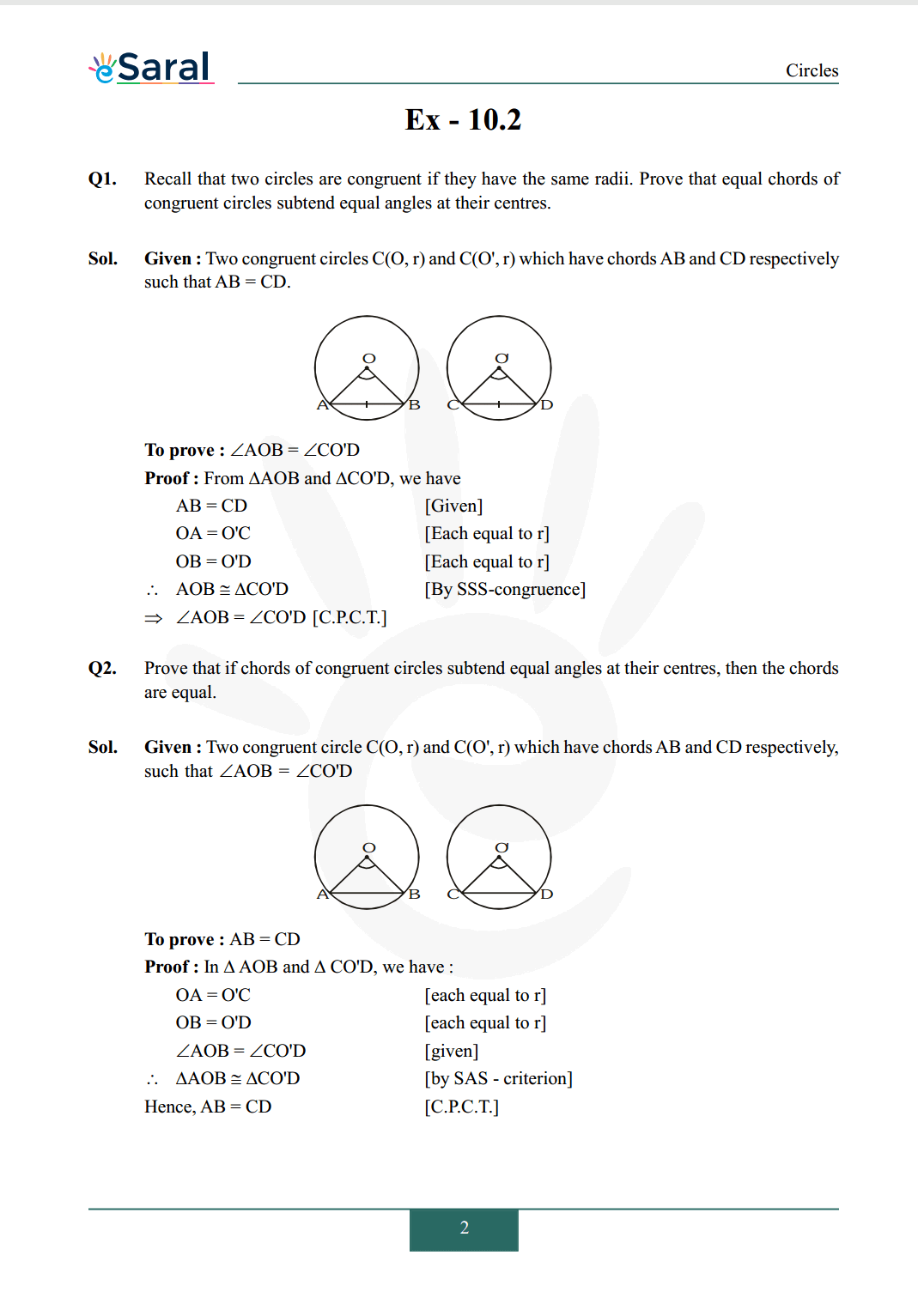Download the eSaral app and start learning from Kota's top IITians and doctors.

# NCERT Solutions for Class 9 Maths chapter 10 Exercise 10.2 - Circles - Free PDF DownloadHey, are you a class 9 Student and Looking for Ways to Download NCERT Solutions for Class 9 Maths chapter 10 Exercise 10.2? If Yes then you are at the right place.

Here we have listed Class 9 maths chapter 10 exercise 10.2 solutions in PDF that is prepared by Kota’s top IITian’s Faculties by keeping Simplicity in mind.

If you want to score high in your class 9 Maths Exam then it is very important for you to have a good knowledge of all the important topics, so to learn and practice those topics you can use eSaral NCERT Solutions.

So, without wasting more time Let’s start.

### Download The PDF of NCERT Solutions for Class 9 Maths chapter 10 Exercise 10.2 "Circles"#### All Questions of Chapter 10 Exercise 10.2

Once you complete the chapter 10 then you can revise Ex. 10.2 by solving following questions

Q1. Recall that two circles are congruent if they have the same radii. Prove that equal chords of congruent circles subtend equal angles at their centres.

Q2. Prove that if chords of congruent circles subtend equal angles at their centres, then the chords are equal.

NCERT Class 9 Maths Book Free PDF

NCERT Class 9 Maths Exemplar Free PDF

Complete Solutions for Class 9 Maths chapter 10

Class 9 Maths Chapter 9 Exercise 9.1 Solutions

Class 9 Maths Chapter 9 Exercise 9.2 Solutions

Class 9 Maths Chapter 9 Exercise 9.3 Solutions

If you have any Confusion related to NCERT Solutions for Class 9 Maths chapter 10 Exercise 10.2 then feel free to ask in the comments section down below.

To watch Free Learning Videos on Class 9 by Kota’s top Faculties Install the eSaral App